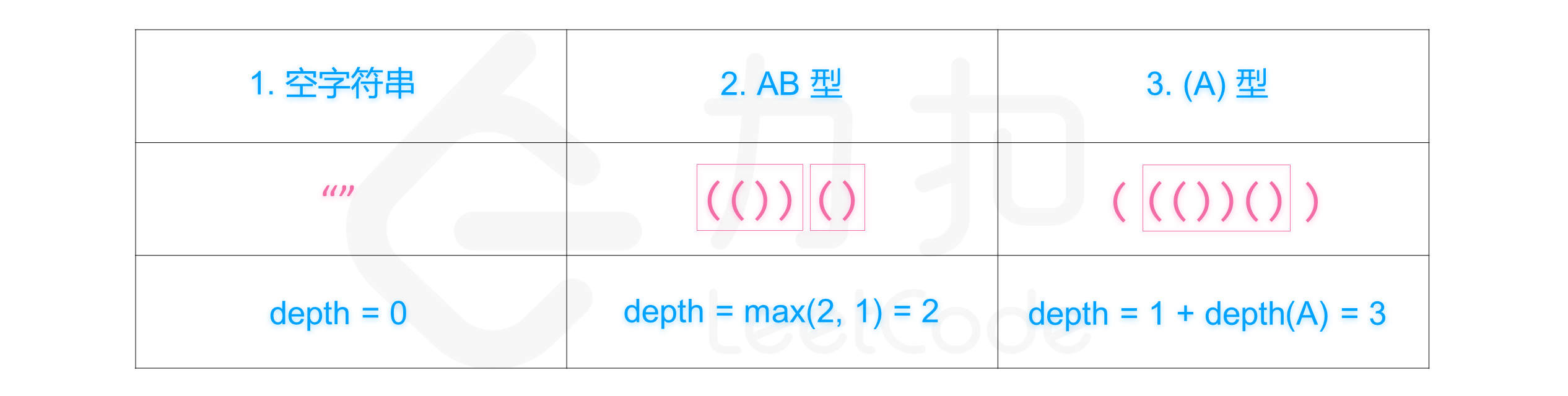# 有效括号的嵌套深度

## 1111. 有效括号的嵌套深度 (Medium)• 不相交：每个 `seq[i]` 只能分给 `A``B` 二者中的一个，不能既属于 `A` 也属于 `B`
• `A``B` 中的元素在原字符串中可以不连续。
• `A.length + B.length = seq.length`
• 深度最小：`max(depth(A), depth(B))` 的可能取值最小。

• `answer[i] = 0``seq[i]` 分给 `A`
• `answer[i] = 1``seq[i]` 分给 `B`

```输入：seq = "(()())"

```

```输入：seq = "()(())()"

```

• `1 < seq.size <= 10000`

```仅由 `"("` 和 `")"` 构成的字符串，对于每个左括号，都能找到与之对应的右括号，反之亦然。

1. 空字符串
2. 连接，可以记作 `AB`（`A` 与 `B` 连接），其中 `A` 和 `B` 都是有效括号字符串
3. 嵌套，可以记作 `(A)`，其中 `A` 是有效括号字符串
```

```类似地，我们可以定义任意有效括号字符串 `s` 的 嵌套深度 `depth(S)`：

1.` s` 为空时，`depth("") = 0`
`  2. s` 为 `A` 与 `B` 连接时，`depth(A + B) = max(depth(A), depth(B))`，其中 `A` 和 `B` 都是有效括号字符串
`  3. s` 为嵌套情况，`depth("(" + A + ")") = 1 + depth(A)`，其中 `A` 是有效括号字符串

```

[] [字符串]